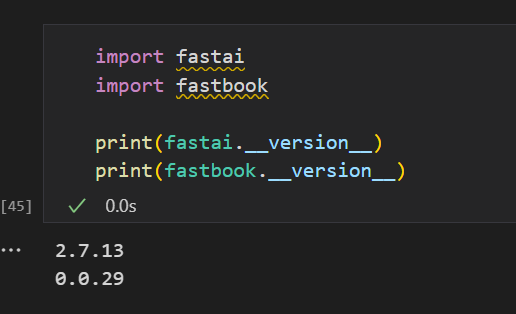# Confusion Matrix for Oxford-IIIT Pet Dataset Classification with fastai

I’m working on Oxford-IIIT Pet Dataset Classification with fastai.
( dataset link - Visual Geometry Group - University of Oxford )

trying to plot the confusion matrix for different dogs categories –

confusion matrix image is looking garbled,

is there any way to resize it so that it is easily interpretable ?I don’t know if it works on notebooks but give it a try (put it before any call to `plot_` functions)

``````figrc= {'figsize': [9,9]} # in inches, increase if needed
matplotlib.rc('figure', **figrc)
``````
2 Likes

You can pass `figsize` and anything else to matplotlib as part of the kwargs when calling `plot_confusion_matrix`

2 Likes